# Two wooden members of 80 × 120-mm uniform rectangular cross section are joined by the simple glued scarf splice shown, Knowing that β = 22° and that the maximum allowable stresses in the joint are, respectively 400 kPa in tension (perpendicular to the splice) and 600 kPa in shear (parallel to the splice), determine the largest centric load P that can be applied.

Question-AnswerCategory: Strength of MaterialsTwo wooden members of 80 × 120-mm uniform rectangular cross section are joined by the simple glued scarf splice shown, Knowing that β = 22° and that the maximum allowable stresses in the joint are, respectively 400 kPa in tension (perpendicular to the splice) and 600 kPa in shear (parallel to the splice), determine the largest centric load P that can be applied.

Two wooden members of 80 × 120-mm uniform rectangular cross section are joined by the simple glued scarf splice shown, Knowing that β = 22° and that the maximum allowable stresses in the joint are, respectively 400 kPa in tension (perpendicular to the splice) and 600 kPa in shear (parallel to the splice), determine the largest centric load P that can be applied.Fig.14. 80

Step No: 1

Draw the force distribution of forces on the splice.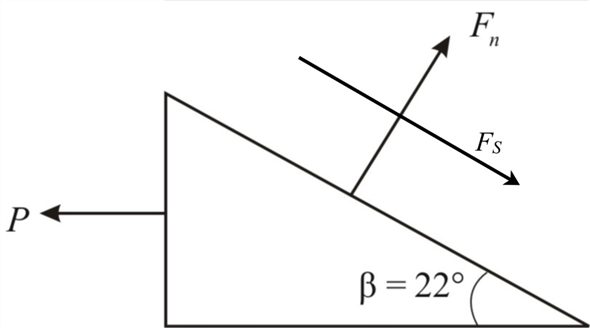Step No: 2

Calculate the area of the inclined plane by using the equation: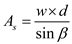Here,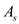is the area of the inclined plane,is the width of the rectangular cross-section,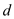is the depth of the rectangular cross-section andis the angle of inclination of the plane.
Substituteforandfor.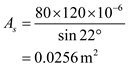Step No: 3

Apply the equilibrium equation along the inclined plane.Substitutefor.Here,is the force along the inclined plane.

Step No: 4

Calculate the Shear stress by using the equation: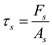Here,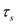is the Shear stress.
Substitute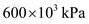for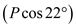for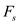and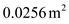for.Step No: 5

Apply the equilibrium perpendicular to the inclined plane.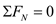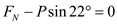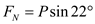Here,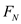is the force perpendicular to the inclined plane.

Step No: 6

Calculate the normal stress by using the equation: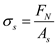Here,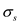is the normal stress.
Substituteforforandfor.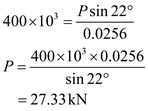Therefore, as per design the allowable value of P is the smaller value: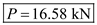.